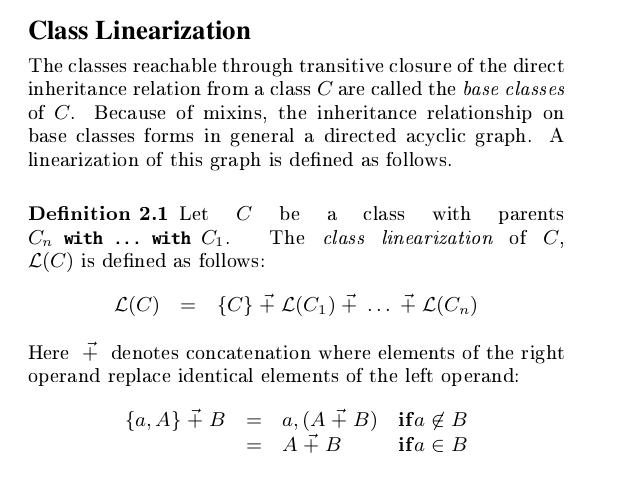I am reading this paper for seminaryso if C extends Cn with Cn-1 with … with C1
the linearization is C → L{C1} → … → L{Cn}
where L(Ci) means linearization of Ci

Under definition 2.3
we read that definition M in Ci overrides M` in Cj if i precedes j in linearization (i < j) (so its intuitive subclass overrides parent class)

so I dont understand definition 2.1 because it seems to contradict this saying that in concatenation right operand replace identical element of the left operand. Can someone explain this to me?

Maybe an example will help? Given:

``````trait A {
def foo: Int = 1
}
trait B extends A {
override def foo: Int = 2
}
trait C extends A {
override def foo: Int = 3
}
class X extends B with C
``````

Then we have by definition 2.1:

``````L(B) = B, A, Object
L(C) = C, A, Object
L(X) = L(C) + L(B)
= C, B, A, Object // only the last occurences of A and Object are kept
``````

And combined with definition 2.3 we can deduce that `(new X).foo` will print `3`.

For an alternative description of linearization and overriding rules, see my brand new paper at the 2021 Scala Symposium: Pathless Scala: A Calculus for the Rest of Scala

3 Likes

My understanding is that we can deduce:

``````L(X) = {X} + L(C) + L(B) = {X, C, A, Object} + L(B) = (now {A, Object} is replaced by elements in L(B) so) {X, C, B, A, Object}
``````

Yeah, I missed the initial X in my example.